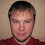## Friday, January 29, 2010

### Overcoming Type Erasure in Matching 2 (Variance)

A commenter (Brian Howard) on the post Overcoming Type Erasure in Matching 1 made a very good point:

Is there a way to deal with some type arguments being contravariant? Try the following:

class A

class B extends A

val AAFunction = new Def[Function1[A,A]]

((a:A) => a) match {case AAFunction(f) => Some(f(new A)); case _ => None} // this is OK

((a:A) => new B) match {case AAFunction(f) => Some(f(new A)); case _ => None} // this is OK

((b:B) => b) match {case AAFunction(f) => Some(f(new A)); case _ => None} // gives a ClassCastException, since new A is not a B

There is a way to do this, however the information is not captured in the Manifest. A manifest is not designed to do full reflection it is by design very light and has little impact on performance. So to provide the functionality requested by Brian one needs to add that information to the Extractor Definition. Have have a possible solution below.
`scala> class Adefined class Ascala> class B extends Adefined class Bscala> object Variance extends Enumeration {     |     val Co, Contra, No = Value     | }defined module Variancescala> import Variance._import Variance._scala> class Def[C](variance:Variance.Value*)(implicit desired : Manifest[C]) {     |     def unapply[X](c : X)(implicit m : Manifest[X]) : Option[C] = {     |         val typeArgsTriplet = desired.typeArguments.zip(m.typeArguments).     |                                                     zipWithIndex     |         def sameArgs = typeArgsTriplet forall {     |             case ((desired,actual),index) if(getVariance(index) == Contra) =>      |                 desired <:< actual      |             case ((desired,actual),index) if(getVariance(index) == No) =>      |                 desired == actual      |             case ((desired,actual),index) =>      |                 desired >:> actual     |         }     |              |         val isAssignable = desired.erasure.isAssignableFrom(m.erasure) || (desired >:> m)     |         if (isAssignable && sameArgs) Some(c.asInstanceOf[C])     |         else None     |     }     |     def getVariance(i:Int) = if(variance.length > i) variance(i) else No     | }defined class Defscala> val AAFunction = new Def[Function1[A,A]]AAFunction: Def[(A) => A] = Def@64a65760scala> ((a:A) => a) match {case AAFunction(f) => Some(f(new A)); case _ => None}res0: Option[A] = Some(A@1bd4f279)scala> ((a:A) => new B) match {case AAFunction(f) => Some(f(new A)); case _ => None}res1: Option[A] = Nonescala> ((b:B) => b) match {case AAFunction(f) => Some(f(new A)); case _ => None}res2: Option[A] = Nonescala> val BAFunction = new Def[Function1[B,A]](Contra,Co)BAFunction: Def[(B) => A] = Def@26114629scala> ((b:A) => b) match {case BAFunction(f) => Some(f(new B)); case _ => None}res3: Option[A] = Some(B@15dcc3ca)`

1.val BAFunction = new Def[Function1[B,A]](Contra,Co)

f:Any = ((b:A) => b)
f match {....} - won't match coz of type "Any"

2.I agree. We're sadly still dependent on compile time types here.

val x:Any = List(1,2,3)
x match {
case IntList(l) => println( s"Match \${l(1)}" );
case _ => println( s"No match" )
}

gives "No match"